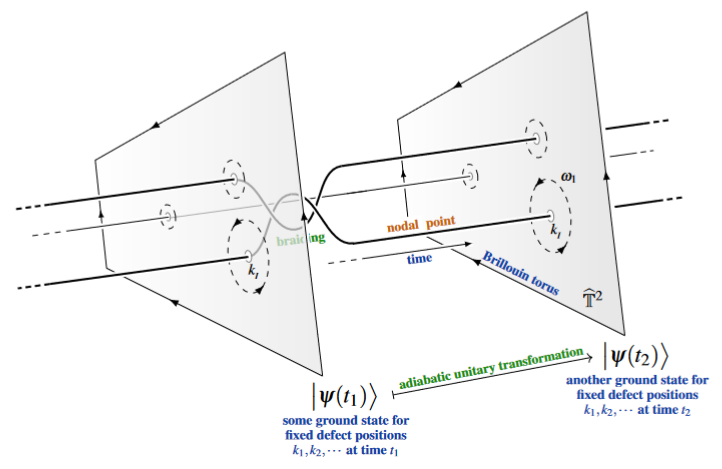Contents

# Contents

## Idea

In quantum physics, the quantum adiabatic theorem for a parameterized quantum system says that under a sufficiently slow motion of the external parameters along a path $\gamma \,\colon\, [0,1] \to P$ in parameter space $P$, eigenstates for mutually commuting quantum observables of the quantum system at $\gamma(0)$ will approximately evolve to eigenstates at $\gamma(1)$, even if the corresponding eigenvalues change significantly.

For adiabatic transport along a loop, $\gamma(0) = \gamma(1)$, this implies that the eigenspaces of the system are acted on by unitary operators, which (at least for multiplicity/eigen-dimension 1) are known as Berry phases.

The adiabatic parameter-action on ground states of (topologically ordered) quantum materials is one model for quantum computation, see at adiabatic quantum computation. If these adiabatic quantum gates depend only on the isotopy class of the parameter path, then one speaks of topological quantum computation (made explicit in Arovas, Schrieffer, Wilczek & Zee 1985, p. 1, Freedman, Kitaev, Larsen & Wang 2003, pp. 6 and Nayak, Simon, Stern & Freedman 2008, §II.A.2 (p. 6), see also at braiding of anyonic defects).

The following graphics is meant to illustrate this general idea for the case of adiabatic transformation along the braiding of nodal points in the Brillouin torus of semi-metal quantum materials (see the discussion at braid group statisticsanyonic band nodes):(graphics from SS22)

### General

The original formulation:

Making explicitbthe unitary action by non-abelian Berry phases: